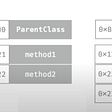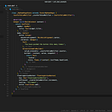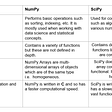# Python under the hood — tips and tricks from a C++-programmers’ perspective 03

`# list -the same for sets (use {} ) and tuples (use () )a = [1, 2, 3]b = [3, 4, 5]c = [*a, *b]print("a = ", a) # [1, 2, 3]print("b = ", b) # [3, 4, 5]print("c = ", c) # [1, 2, 3, 3, 4, 5]`
`# non-keyword argumentst = (1, 2, 3)def tt(*args):for arg in args:   print(arg+1)       tt(*t) # 2, 3, 4###### keyword argumentsd = {'a' : 1, 'b' : 2}def dd(**kwargs):   for key, value in kwargs.items():      print("Key: ", key, " value: ", value)dd(**d)  # Prints: Key:  a  value:  1#         Key:  b  value:  2`
`r = 5 if True else 4 # 5`
`l = list(range(10))l_even = [x for x in l if x%2 == 0]s_even_sq = {x**2 for x in l if x%2 == 0}print("l = ", l) # [0, 1, 2, 3, 4, 5, 6, 7, 8, 9]print("l_even = ", l_even) # [0, 2, 4, 6, 8]print("s_even_sq = ", s_even_sq) # {0, 64, 4, 36, 16}`
`a = 1b = 1c = aprint("a == b :", a == b) # Trueprint("a == c :", a == c) # Trueprint("a is b :", a is b) # Trueprint("a is c :", a is c) # True######x = [1, 2, 3, 4, 5]y = [1, 2, 3, 4, 5]z = xprint("x == y :", x == y) # Trueprint("x == z :", x == z) # Trueprint("x is y :", x is y, "<--") # Falseprint("x is z :", x is z) # True`

--

--

--

## More from SmartLab AI

Deep Learning and AI solutions from Budapest University of Technology and Economics. http://smartlab.tmit.bme.hu/

Love podcasts or audiobooks? Learn on the go with our new app.

## Swift method dispatch## Flash Stock Firmware on Samsung Galaxy J2 SM-J200GU## Glow — Local MQTT## Qiskit Camp 101## Ansible With AWS## Is it easy to test a Flutter widget?## How To Navigate The Linux Filesystem?## SmartLab AI

Deep Learning and AI solutions from Budapest University of Technology and Economics. http://smartlab.tmit.bme.hu/

## 3 Methods for Handling Missing Values with Python## SciPy-Tutorial## Two Ways to Immediately Improve Your Python Code## Tuple in Python : Creating, Accessing Tuple